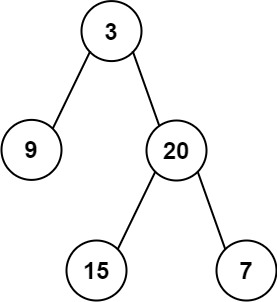| English | 简体中文 |

# 105. Construct Binary Tree from Preorder and Inorder Traversal

## Description

Given two integer arrays preorder and inorder where preorder is the preorder traversal of a binary tree and inorder is the inorder traversal of the same tree, construct and return the binary tree.

Example 1:Input: preorder = [3,9,20,15,7], inorder = [9,3,15,20,7]
Output: [3,9,20,null,null,15,7]


Example 2:

Input: preorder = [-1], inorder = [-1]
Output: [-1]


Constraints:

• 1 <= preorder.length <= 3000
• inorder.length == preorder.length
• -3000 <= preorder[i], inorder[i] <= 3000
• preorder and inorder consist of unique values.
• Each value of inorder also appears in preorder.
• preorder is guaranteed to be the preorder traversal of the tree.
• inorder is guaranteed to be the inorder traversal of the tree.